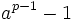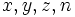# Difference between revisions of "Fermat (mathematics)"

### In number theory

Fermat's little theorem: Ifis a prime number andis an integer that is not a multiple of, thendivides.

Primary subject wiki entry: Number:Fermat's little theorem.

Fermat's last theorem: The equation:has no positive integer solutions forwith.

Primary subject wiki entry: Number:Fermat's last theorem.

Fermat number: This is a natural number of the formwhereis a nonnegative integer.

Primary subject wiki entry: Number:Fermat number.

Other subject wiki entries: Groupprops:Fermat number.

Fermat prime: This is a prime number of the form, whereis a nonnegative integer.

Primary subject wiki entry: Number:Fermat prime.

Other subject wiki entries: Groupprops:Fermat prime.

Fermat pseudoprime: A composite natural numberis termed a Fermat pseudoprime to base, for an integer, ifandare relatively prime andis a multiple of.

Primary subject wiki entry: Number:Fermat pseudoprime.